Do you like the article?
Share it with others -

Use new possibilities of MetaTrader 5

# Break Through The Strategy Tester Limit On Testing Hedge EA

30 August 2007, 11:07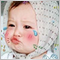7
14 124

### Introduction

An idea of testing hedge EA in the strategy tester will be given in this article. As you know, the strategy tester has its own limit unabling to open any order for another symbol. Every user that would like to test his/her own hedge Expert Advisors needs to test it/them live only. But does this limit our capabilities? I am sure that every hedge trader needs to test his own EA before trading live. So, I am giving the idea of generating a virtual strategy testing behavior (tester-like) to you all with hope that it can help us break through the mt4 strategy tester limit and hope that it will useful for further usage.

### The Concept Of Virtual Tester

The idea of a virtual tester appeared in my head while working with "Files" function in mq4. An idea of taking some important data from a file to set a virtual trading scheme has come out of my brain, "Can this be an answer of testing my hedge EA ?" Let's try it out.

My virtual tester does not need any other external program or software. Everything can be done by means of mq4 parameters. The concept of this virtual tester is to let the indicated parameters of opening or closing hedge orders tell us to collect the necessary data. Such as opening price, opening time, closing price, closing time and all other important data. After the necessary data is set, they will be used for comparing with the latest tick value of any compatible type, such as open price and last Bid, or open price and last Ask. Such values can lead us to the profit calculation method that will guide us to collect a new group of data after they meet the closing hedge condition.

These groups of data will be exported to a file for the further usage. After the testing is done and all data types are collected to a file(s), we will be able to see "how the hedge EA is performed ". By getting the data from these files to plot them as an indicator of the performance's curve, I think we can finish the virtual testing of our hedge EA.

By this concept, I assume that we can get the testing result that is similar to the real strategy tester result. By the way, this is only an idea of creating a tester for hedge Expert Advisors. I will not guarantee that it will be exactly the same as a real tester. But hope that it will be a good reference for future usage.

Now let's begin.

### The Easy Meaning Of Hedge Trading

Before we start, let's talk a little about "HEDGE".

The easy way to say about hedging is to open two opposite trades of 2 currency pairs at the same time. This is to lower the trading risk, if one moves up, one will surely move down, but there is nothing to worry about, because we’ve got both buy and sell orders at the same time, then even if we lose one, we get one, that is why they call it ”low risk”. There are many kinds of opposite trade styles in the Forex world.

• For 2 currency pairs that always move the same way like EURUSD and GBPUSD, opening buy-EURUSD and sell-GBPUSD at the same time is hedging.
• For 2 currency pairs that always move the opposite way like EURUSD and USDCHF, opening buy-EURUSD and buy-USDCHF is to hedge them too.
• Or even open both buy and sell EURUSD at the same time is to hedge too, but this is called “arbitrate” sometimes.

In hedge trading there are some facts that can not be doubted.

1. The Correlation : is the statistical measure of the relationship between two currencies. The correlation coefficient ranges between -1 and +1. A correlation of +1 implies that the two currency pairs will move in the same direction 100% of the time. A correlation of -1 implies the two currency pairs will move in the opposite direction 100% of the time. A correlation of zero implies that the relationship between the currency pairs is completely random (read more here).
2. The Lot Size Ratio : To trade 2 currency pairs that neither move the same way nor opposite way, the lot ratio is quite necessary, because their own volatility and movement capability are different like turtle and rabbit, if one is a turtle then another one will be the rabbit. Lot ratio can lower the risk, affected by more powerful movement pair or rabbit pair, by placing the higher lot size to the turtle pair to guarantee the less loss when the rabbit pair is in a negative move. Then you can gain more from positive turtle, or in the other word, you can replace that negative profit from the loss-rabbit by the gain-turtle. And thus hedging technique can ensure you that you will not lose more than opening only one trade in negative side.

By the way, have you ever wondered, how a hedger gains from that trading style. Don’t worry, there will always be an overlap between the 2 currency pairs, the correlation is not a constant scheme in nature, there will frequently be a delay by one pair, one starts moving, then one will follow after it, again: like rabbit and turtle - a rabbit will take a rest, wait for the turtle to beat and win. That’s why hedgers gain a nice profit from them. And nowadays many people use hedging style to make money in Forex, nothing to worry about. Hedge, wait, close when a positive profit is shown. That’s it.

### The Hedge Concept

Before starting to code the virtual tester, let's try to understand the hedging concept in an experiment. Without the hedging concept we will never know which type of data should be exported, recorded and calculated. These data can show us which type of order should be virtually generated. In this experiment I will set the hedging rules like this.

• Open hedge daily at the beginning of the day
• Close when it reaches \$100 (take the lot size of 1 and 2)
• Collect the tick price data hourly***
• Clear them daily when the new day begins even if they did not reach the target profit
• Buy only EURJPY 2 lots and sell GBPJPY 1 lot

According to these rules the virtual orders need daily open prices (of both pairs) be used as an order-open-price. To calculate the intra-day profit, the price on every hour, as tick price, of every day should be recorded as the data for the order-close-price (ask for sell and bid for buy) and should be recorded together with the tick's time( to make sure that the tick price is from the same time value). And due to the concept of opening the hedge daily, I will separate all data needed into 2 file-type, that are daily-open and tick-value of both 2 pairs. Both 2 data types will be exported as a string file(s) with separate name, like GBPJPYD1.csv and GBPJPYTick.csv.

And because of tick data that we wanted the virtual tester to be as much similar to the real one as possible, these 2 steps have to be proceeded.

• Making a script to export the daily open price of GBPJPY into a file
• Making a script to export the daily tick price of GBPJPY into a file

And both 2 steps should be done for EURJPY too.

But I think we can combine them into one expert advisor, this EA should export both 2 types of data into 2 separate files. Then after this EA finished the data recording process, a new one EA for generating the virtual trading will get all data of both GBPJPY and EURJPY from all exported files to perform the virtual testing show.

### The 3 Steps To Break The Testing Limit

By the idea above, I have concluded that our dream to break through this limit could be done by the following 3 steps.

1. Take the price data and output them into some files using an EA.
2. Generate the virtual trading with another separate EA that exports the result as a file too.
3. Review the result as an indicator in separate window.

So let's begin the first step.

### Step 1: Export The Price Data

Below is the Expert Advisor to export the daily-open-price of attached symbol into a file, named "GBPJPYD1.csv" for GBPJPY and "EURJPYD1.csv" for EURJPY, in the mean time it will export the tick price into a file too, named like this "symbolT. csv" (the same as D1 file). Please read comments to learn how the EA works.

Note : All the files created by this EA will be exported to "MetaTrader 4/tester/files" directory.

```//+------------------------------------------------------------------+
//|                                                    symbol-D1.mq4 |
//|                                  http://pipsmaker.wordpress.com/ |
//+------------------------------------------------------------------+
#include <stdlib.mqh>

extern string StartDate = "2007.03.17"   //set the starting date to get the same time data
,StopDate  = "2007.06.28";  //set the testing time limit to protect the over
//data recording process

extern bool For_OP_SELL = true;/*This is to guide for the right type of data to be collected
->if For_OP_SELL = true
the daily Open will be collected as an order open price
->if For_OP_SELL = false , means for OP_BUY,
the daily Open+SPREAD will be collected instate.
*/

string name,tname;
//+------------------------------------------------------------------+
//| expert initialization function                                   |
//+------------------------------------------------------------------+
int init()
{
//----

//----
return(0);
}
//+------------------------------------------------------------------+
//| expert deinitialization function                                 |
//+------------------------------------------------------------------+
int deinit()
{
//----

//----
return(0);
}
//-------------------------------------------------------------------+
//| Some Essential Parameters In This EA                             |
//-------------------------------------------------------------------+
int day                   // a variable to mark that today Open has been collected
,ho                    // handle of the file recording the Open price
,ht                    // handle of the file recording the tick price
,x=1                   /* the order of D1 file increasing every time D1 data is
equal to 4086 characters and generate the new recording file*/
,xt=1                  // same as x but for tick data
,bartime               // a variable to mark that current bar's Open has been collected
;
double ot                 // latest Open Time
,op                 // latest Open price
,lt                 // latest tick time
,ltk                // latest tick price
;
string OStr               // the string to collect the daily open
,TStr               // the string to collect the tick value
;
//+------------------------------------------------------------------+
//| expert start function                                            |
//+------------------------------------------------------------------+
int start()
{

/*---------------------Only collect the data in the specific time period.----------------------*/
if(TimeToStr(TimeCurrent(),TIME_DATE)>=StartDate && TimeToStr(TimeCurrent(),TIME_DATE)<=StopDate)
{
name=Symbol()+x+"D1.csv";       // setup the name of daily open price file
tname=Symbol()+xt+"T.csv"       // the name of tick price file
;
//----
if(day!=TimeDay(Time))        // the new day has come
{
ot=Time;                   // get the new bar time
if(For_OP_SELL)op=Open;    // get the new order open price for SELL Symbol

// else if collecting for the BUY Symbol

OStr=OStr+TimeToStr(Time,TIME_DATE)+",";//collect the new time data separate each data with ","
OStr=OStr+DoubleToStr(op,Digits)+",";      //now the new open price

//~~~~~~~~~~~~~~~~~~~~~~~~~~~~~~~~~~~~~~~~~~now it's time to export as a file

ho=FileOpen(name ,FILE_CSV|FILE_WRITE);  // open a file to record the daily open
if(ho>0)                                 // if the file Symbol()+x+"D1.csv" exist
{
FileWrite(ho,OStr);                     // write the collected data
FileClose(ho);                          // close the file every time your file process done
if(StringLen(OStr)==4086){x++;OStr="";} /* if the data contains 4086 characters set new x to*/
}                                        /*   create the new file and prepare the new string for
the new file
*/

Print(TimeToStr(Time,TIME_DATE));     // print the collected day time

int thex=FileOpen(Symbol()+"x.csv",FILE_CSV|FILE_WRITE);
// create a file to record "how many x?" for D1 file for further usage
if(thex>0)                               // if the file exist
{
string xs=DoubleToStr(x,0);             // transform x into string
FileWrite(thex,xs);                     // write the x value
FileClose(thex);                        // close file (every time you finish)
}

day=TimeDay(Time);                   // now mark today as collected
}

//--------------------------------FOR TICK VALUE
/*Because of the decision of collecting the tick data hourly*/
if(bartime!=Time)                     // the new hourly bar has come
// and to make it more flexible when you decided
// to collect data in another time frame
{
lt=TimeCurrent();                      // get the tick time

if(!For_OP_SELL) ltk=Bid;              // the tick price for sell order

TStr=TStr+TimeToStr(lt,TIME_DATE|TIME_MINUTES)+",";
// insert the data into the collected string
TStr=TStr+DoubleToStr(ltk,Digits)+","; //

//~~~~~~~~                               // now export the data
ht=FileOpen(tname,FILE_CSV|FILE_WRITE);  // open a file to record the tick value
if(ht>0)                                 // if the file Symbol+xt+"T.csv" exist
{
FileWrite(ht,TStr);                     // write the collected tick with time
FileClose(ht);                          // finish.
if(StringLen(TStr)==4080){xt++;TStr="";}// prepare for new file if this file reached 4080 character
}

int thext=FileOpen(Symbol()+"xt.csv",FILE_CSV|FILE_WRITE);
// record the xt value .. same as D1 file

if(thext>0)                              // if the file exist
{
string xts=DoubleToStr(xt,0);           // transform into string
FileWrite(thext,xts);                   // write xt
FileClose(thext);                       // done
}
bartime=Time;                           // mark as current hourly bar Open price has been collected
}
}
else if(TimeToStr(TimeCurrent(),TIME_DATE)>StopDate)  // if out of specified period
Print("Done.");                                       // let us know it all done.
//----
return(0);
}
//+------------------------------------------------------------------+```

### Step 2: Generate The Virtual Trading

This step is the most excited step. The step of making the hedge EA to be testable by the strategy tester. See the script below to know what it looks like. And don't forget to read comments to understand how it works. And like in the first EA, the result file will be exported to "MetaTrader 4/tester/files" directory.

```//|                                                 VirtualHedge.mq4 |
//|                                  http://pipsmaker.wordpress.com/ |
//+------------------------------------------------------------------+
#include <stdlib.mqh>

extern string  StartDate         = "2007.03.17";    // specify the testing period the same as collected data
extern string  StopDate          = "2007.06.27";
extern string  BaseSymbol        = "GBPJPY";        // the base symbol
extern string  HedgeSymbol       = "EURJPY";        // the hedge symbol

extern int     Base_OP           = OP_SELL;         // by the rules always sell GBPJPY

extern double  BaseLotSize       = 1.0;             // take the lot size of 1 for GBPJPY
extern double  HedgeLotSize      = 2.0;             // and take 2 lots for EURJPY

extern double  ExpectProfit\$     = 100;             // always close when reach \$100

extern bool    PrintDetails      = true;            // print the order opening and closing detail ?

int BSP                                      // the spread of GBPJPY
,HSP                                      // the spread of EURJPY
,BOP=-1                                   // OrderType of GBPJPY
,HOP=-1                                   // OrderType of EURJPY
,day=0                                    // for marking that today hedge was executed
,hr                                       // handle of the file for exporting the hedge result
,p=1
,BC                                       // the base-symbol's contract size
,HC                                       // the hedge-symbol's contract size
,floating=0                               // for marking as there are some orders currently floating
,Pointer=0                                // the Pointer of each string data
,AL                                       // Account Leverage
,on                                       // order number
;
double BOpen                                 // open price of base symbol
,HOpen                                 // open price of hedge symbol
,BLots                                 // base symbol lotsize
,HLots                                 // base symbol lotsize
,lastTick                              // to mark the last tick for calculating the current profit
,BPF                                   // base symbol order profit
,HPF                                   // hedge symbol order profit
,TPF                                   // the total profit
,CurBalance                            // the array to collect the hedge results
,CurB=0                                // the current balance
,BTick                                 // Base tick
,HTick                                 // Hedge tick
,BD1Time                               // Base daily open time
,HD1Time                               // Hedge daily open time
,BTTime                                // Base tick time
,HTTime                                // Hedge tick time
;

string CurTrade                              // a string to show the current performance as a comment
,BORD                                  // a string to specify the type of arrows and text of base symbol
,HORD                                  // same thing but for hedge symbol
,hobstr                                // the whole string of the base symbol open price data
,bstr                                  // the string of all daily data of base Symbol
,hstr                                  // the string of all daily data of hedge Symbol
,btstr                                 // the string of tick data of base Symbol
,htstr                                 // the string of tick data of hedge Symbol
,pstr                                  // the string for exporting result data
;

color SELLCL=DeepSkyBlue                     // the color of arrows to mark that sell order was executed
,BUYCL=HotPink                          // the color of arrows to mark that buy order was executed
,BCL                                    // the color of base symbol, varies by the Base_OP parameter
,HCL                                    // the color of hedge symbol, varies by the Hedge_OP parameter
;

bool closed=true                             // for marking as all trades were closed
,trimb=true                             // get rid of taken data of base Symbol or not?
,trimh=true                             // get rid of taken data of hedge Symbol or not?
,trimbd1=true                           // get rid of taken daily data of base Symbol or not?
,trimhd1=true                           // get rid of taken daily data of hedge Symbol or not?
;
//+------------------------------------------------------------------+
//| expert initialization function                                   |
//+------------------------------------------------------------------+
int init()
{
//----
CurBalance=AccountBalance();                      //mark the initial deposit
CurB=AccountBalance();                            //mark the current balance
pstr=pstr+DoubleToStr(CurBalance,2)+",";          //collect the performance string

AL=AccountLeverage();                             //get the Account Leverage

BC =MarketInfo(BaseSymbol  ,MODE_LOTSIZE);        //get the contract size for calculating profit
HC =MarketInfo(HedgeSymbol ,MODE_LOTSIZE);

BOP=Base_OP;                                      //get the OrderType
HOP=Hedge_OP;

BLots=BaseLotSize;                                //get the lot size
HLots=HedgeLotSize;

string RName=BaseSymbol+"_"+HedgeSymbol+"_result"+p+".csv";
//name the performance file
//this file is needed to copy to
/* Program files/MetaTrader 4 /Experts/files */

hr =FileOpen(RName ,FILE_CSV|FILE_WRITE);         //open the file to export the initial deposit

if(hr>0)                                          //if the file exist
{
FileWrite(hr,pstr);                              //export the initial deposit
FileClose(hr);                                   //close file
}

if(Base_OP==OP_SELL){BCL=SELLCL;BORD="sell";}     //specify the parameter leading to create
else                {HCL=SELLCL;HORD="sell";}

getdata(BaseSymbol);                              //get all data of BaseSymbol
getdata(HedgeSymbol);                             //all data of HedgeSymbol
//the function located at the bottom
return(0);
}
//+------------------------------------------------------------------+
//| expert deinitialization function                                 |
//+------------------------------------------------------------------+
int deinit()
{
//----

//----
return(0);
}
//+------------------------------------------------------------------+
//| expert start function                                            |
//+------------------------------------------------------------------+
int start()
{
string RName=BaseSymbol+"_"+HedgeSymbol+"_result"+p+".csv"; //name the performance file

//--------------------------Only perform the show of specified period--------------------------//
if(TimeToStr(TimeCurrent(),TIME_DATE)>=StartDate && TimeToStr(TimeCurrent(),TIME_DATE)<=StopDate)
//mark as no any order now
{
if(day!=TimeDay(Time))                                  //the new day has come
{

{
if(BOpen!=0 && HOpen!=0)                                 //check if yesterday hedge still floating
{

{
BPF=((BTick-BOpen)*BLots*BC)/BOpen;
}
else
{
BPF=((BOpen-BTick)*BLots*BC)/BOpen;
}

{
HPF=((HTick-HOpen)*HLots*HC)/HOpen;
}
else
{
HPF=((HOpen-HTick)*HLots*HC)/HOpen;
}

TPF=BPF+HPF;                                            //the total current profit

CurB+=TPF;

CurBalance=CurB;                                        //get the latest AccountBalance

pstr=pstr+DoubleToStr(CurBalance,2)+",";                //insert into the performance string

floating=0;                                             //mark as no any order now

BOpen=0;                                                // block the profit calculation process
HOpen=0;

if(BOpen==0 && HOpen==0)                                //make sure there is no any order now
{
closed=true;                                           //mark all orders were closed

CreateObject("R : "+on,OBJ_TEXT,Time,Close,0,0,DarkViolet,"","Cleared With Profit Of : "+DoubleToStr(TPF,2));
//create the cleared all orders text

if(PrintDetails)Print("Cleared Hedge With Profit : "+DoubleToStr(TPF,2));
//print latest action if PrintDetails allowed

hr =FileOpen(RName ,FILE_CSV|FILE_WRITE);             //open the result file to prepare for file writing
if(hr>0)
{
FileWrite(hr,pstr);                                  //export the data
FileClose(hr);
}
if(StringLen(pstr)>4086){p++;pstr="";}                //set the new file name if pstr is larger than 4086 characters

int thep=FileOpen("p.csv",FILE_CSV|FILE_WRITE);       //this file is needed to copy to
/* Program files/MetaTrader 4 /Experts/files */
//too

// record the p value as a file

if(thep>0)                                           // if the file exist
{
string ps=DoubleToStr(p,0);                         // transform into string
FileWrite(thep,ps);                                 // write p
FileClose(thep);                                    // done
}

}
}

if(floating==0)                                          //now all yesterday hedge were cleared
{
trimb=true; trimh=true;                                 //allow getting tick data for today to work

//----------GETTING TODAY ORDER OPEN PRICE AND TIME----------//
if(trimbd1)                                              //getting the daily base price allowed
{
Pointer=StringFind(bstr,",",0);                      //find the nearest "," from the beginning of string
BD1Time=StrToTime(StringSubstr(bstr,0,Pointer));     //get the time value located before the "," syntax
bstr=StringSubstr(bstr,Pointer+1,0);                 //trim off the taken data

Pointer=StringFind(bstr,",",0);                      //find the nearest "," from the trimmed data string
BOpen=StrToDouble(StringSubstr(bstr,0,Pointer));     //get the PRICE value located before the "," syntax
bstr=StringSubstr(bstr,Pointer+1,0);                 //trim off the taken data again to prepare for next time
}
if(trimhd1)                                              //getting the daily hedge price allowed
{
Pointer=StringFind(hstr,",",0);                      //all processes are the same as bstr above
HD1Time=StrToTime(StringSubstr(hstr,0,Pointer));     //...
hstr=StringSubstr(hstr,Pointer+1,0);                 //...

Pointer=StringFind(hstr,",",0);                      //...
HOpen=StrToDouble(StringSubstr(hstr,0,Pointer));     //...
hstr=StringSubstr(hstr,Pointer+1,0);                 //...
}
//--------GETTING TODAY ORDER OPEN PRICE AND TIME ENDED--------//

if(BOpen!=0 && HOpen!=0 && CurBalance>(BLots+HLots)*BC/AL)
//make sure the data taken is not zero and margin balance still available
{
floating=1;            //mark as hedge sent

closed=false;          //mark as all orders opened

on++;                  //new hedge orders have opened

if(PrintDetails)      //if PrintDetails allowed
{
Print(on+" Opened : "+BaseSymbol+" "+DoubleToStr(BLots,2)+" lots @ "+DoubleToStr(BOpen,Digits)+".");
Print(on+" Opened : "+HedgeSymbol+" "+DoubleToStr(HLots,2)+" lots @ "+DoubleToStr(HOpen,Digits)+".");
}
}
else                                                 //in case can not send hedge
{
Comment("Can Not Open The Trade : No Margin Available");
}                                                    //let us know

if(closed==false)                                    //hedge sent
{
//create the buy and sell arrow and text
//this function is located at the bottom of the EA
CreateObject("B : "+on,OBJ_ARROW,Time,Open-20*Point,0,0,BCL,BORD,"");

CreateObject("H : "+on,OBJ_ARROW,Time,Open+30*Point,0,0,HCL,HORD,"");
}
}
}

day=TimeDay(Time);                                     //mark as proceeded
}

//-------------------------For Each Tick----------------------------//

if(lastTick!=Hour())                 //the new hour has come
{

if(trimb && StringFind(btstr,",",0)>0)                         //getting base tick allowed
{
Pointer=StringFind(btstr,",",0);                     //same process as getting daily data above
BTTime=StrToTime(StringSubstr(btstr,0,Pointer));
btstr=StringSubstr(btstr,Pointer+1,0);

Pointer=StringFind(btstr,",",0);
BTick=StrToDouble(StringSubstr(btstr,0,Pointer));
btstr=StringSubstr(btstr,Pointer+1,0);
}
if(trimh && StringFind(htstr,",",0)>0)                         //if getting hedge tick allowed
{
Pointer=StringFind(htstr,",",0);
HTTime=StrToTime(StringSubstr(htstr,0,Pointer));
htstr=StringSubstr(htstr,Pointer+1,0);

Pointer=StringFind(htstr,",",0);
HTick=StrToDouble(StringSubstr(htstr,0,Pointer));
htstr=StringSubstr(htstr,Pointer+1,0);
}

if(TimeDay(BD1Time)==TimeDay(BTTime) && TimeDay(HD1Time)==TimeDay(HTTime))
//only if the tick is form the same day
{
trimbd1=true; trimhd1=true;                              //allow to get the next day value

if(TimeHour(BTTime)==TimeHour(HTTime))                   //and same time hour
{

trimb=true; trimh=true;                                //allow to get the next tick value

if(BOpen!=0 && HOpen!=0)                               //if the calculation process allowed
{

{
BPF=((BTick-BOpen)*BLots*BC)/BOpen;                 //the base-symbol's current profit
}
else
{
BPF=((BOpen-BTick)*BLots*BC)/BOpen;
}

{
HPF=((HTick-HOpen)*HLots*HC)/HOpen;                  //the hedge-symbol's current profit
}
else
{
HPF=((HOpen-HTick)*HLots*HC)/HOpen;
}

TPF=BPF+HPF;                                          //the total current profit

if(TPF > ExpectProfit\$)                               //when they need to close
{

BOpen=0;                                             //set the new value of order open price
HOpen=0;                                             //and block the profit calculation process

CurTrade="No Any Hedge Order Now.";                  //Hedge was closed

floating=0;                                          //mark as hedge was closed
CurB+=TPF;

CurBalance=CurB;                                     //get the last balance equity

pstr=pstr+DoubleToStr(CurBalance,2)+",";             //insert into performance string

CreateObject("R : "+on,OBJ_TEXT,Time,Close,0,0,YellowGreen,"",
"Close With Profit Of : "+DoubleToStr(TPF,2));
//create the closed text

if(PrintDetails)                                     //Print the last Close detail
{
Print(on+" Closed "+BaseSymbol+" @ "+DoubleToStr(BTick,Digits));
Print(on+" Closed "+HedgeSymbol+" @ "+DoubleToStr(HTick,Digits));
Print(on+" Closed Hedge With Profit : "+DoubleToStr(TPF,2));
}
hr =FileOpen(RName ,FILE_CSV|FILE_WRITE);    //open it again to prepair for file writing

if(hr>0)
{
FileWrite(hr,pstr);                         //export the data
FileClose(hr);
}

if(StringLen(pstr)>4086){p++;pstr="";}        //set the new file name if pstr is larger than 4086 characters

thep=FileOpen("p.csv",FILE_CSV|FILE_WRITE);
// record the p value again

if(thep>0)                                   // if the file exist
{
ps=DoubleToStr(p,0);                        // transform into string
FileWrite(thep,ps);                         // write p
FileClose(thep);                            // done
}

}
}

}
else                                             //in case the data values are not from the same tick time
{
if(BTTime>HTTime){trimb=false;}                //freeze the offside data to can not be trim off

else             {trimh=false;}
}
}
else                                              //in case tick data is offside from today
{
if(BTTime>BD1Time){trimb=false;}               //freeze the offside data to can not be trim off
else
if(BTTime<BD1Time){trimbd1=false;}

if(HTTime>HD1Time){trimh=false;}
else
if(HTTime<HD1Time){trimhd1=false;}
}
}

lastTick=Hour();                                     //mark as latest tick proceeded
}

Comment("\nBOpen : "+DoubleToStr(BOpen,Digits)    //show the current situation
,"\nHOpen : "+DoubleToStr(HOpen,Digits)
,"\nBOT : "+TimeToStr(BD1Time,TIME_DATE)
,"\nHOT : "+TimeToStr(HD1Time,TIME_DATE)
,"\nBTick : "+DoubleToStr(BTick,Digits)
,"\nHTick : "+DoubleToStr(HTick,Digits)
,"\nBTT : "+TimeToStr(BTTime,TIME_DATE|TIME_MINUTES)
,"\nHTT : "+TimeToStr(HTTime,TIME_DATE|TIME_MINUTES)
,"\nfloating : "+floating
,"\nclosed : "+closed
,"\ntrimb : "+trimb
,"\ntrimh : "+trimh
,"\n"
,"\nCurOrderNo. : "+on
,"\nCurBalance : "+DoubleToStr(CurBalance,2)
);
//----
return(0);                                               //ALL DONE.
}
//+------------------------------------------------------------------+
//| A Function To Make This Virtual Tester Looks Like The Real One   |
//+------------------------------------------------------------------+
void CreateObject(string name,int type, int time1, double price1, int time2,
double price2, color cl,string ordtype,string txt)
{

if(type==OBJ_TREND)
{
ObjectCreate(name,type,0,time1,price1,time2,price2);
ObjectSet(name,OBJPROP_COLOR,HotPink);
}

if(type==OBJ_ARROW)
{
ObjectCreate(name,type,0,time1,price1);
ObjectSet(name,OBJPROP_COLOR,cl);
if(ordtype=="sell")ObjectSet(name,OBJPROP_ARROWCODE,221);
else              ObjectSet(name,OBJPROP_ARROWCODE,222);
}

if(type==OBJ_TEXT)
{
ObjectCreate(name,type,0,time1,price1);
ObjectSetText(name,txt,8,"Comic Sans MS",cl);
}
}
//+------------------------------------------------------------------+
//| GETTING ALL DATA FROM FILES FUNCTION                             |
//+------------------------------------------------------------------+
void getdata(string sym)
{
Comment("Collecting Data.","\n\nPlease Wait........");  //let us know that getting data still in process

int x =FileOpen(sym+"x.csv",FILE_CSV|FILE_READ)   //get how many files of D1 and tick
,pter=0,s=0,v=0
,lastME=0,t=0
;
double ME,U;
string str,str2;
,xtc=1
;
FileClose(x);
FileClose(xt);

if(xta>xa)xtc=xta;                                     //make it run only in one for loop
else      xtc=xa;

pter=0;s=0;
for(int i=1;i<=xtc;i++)                                //the loop to get all string data
{
string name=sym+i+"T.csv"                             //set the data file name
,d1  =sym+i+"D1.csv"
;

//------------------------------------------------------------
FileClose(h);

if(sym==BaseSymbol)                                  //if get the data of base symbol
{
btstr=btstr+source;
}
else                                                 //if hedge symbol
{
htstr=htstr+source;
}

//------------------------------------------------------------
{

if(sym==BaseSymbol)                                 //if get base daily data
{
bstr=bstr+d1s;
}
else                                                //if get hedge data
{
hstr=hstr+d1s;
}
}
}
}
//------------------------------------ALL DONE---------------------------------------------//```

### Step 3: Review The Result

After the virtual orders were executed and hedging results have been recorded, we can take those data to show off our hedging concept. To do that, I decided to export all the recorded data as an indicator to plot the performance curve in a separate window as in many indicators like CCI, RSI or ATR and etc. And all the files from the second EA should be copied into "MetaTrader 4/experts/files" directory.

To finish this curve, the indicator below is needed.

```//+------------------------------------------------------------------+
//|                                                  performance.mq4 |
//|                                         http://pipsmaker.wordpress.com/ |
//+------------------------------------------------------------------+

#property indicator_separate_window
#property indicator_buffers 1
#property indicator_color1 Goldenrod
//---- input parameters
extern string    BaseSymbol="GBPJPY";
extern string    HedgeSymbol="EURJPY";
//---- buffers
double ExtMapBuffer1[]                 //this is the indicator buffer
,curve                  //this array is for collecting the result from the performance file
;

int handle;                            //maybe no need to explain anymore
string data;
int len=0
,i=0
,j=0
,p
,pv
,pter=0
;
//+------------------------------------------------------------------+
//| Custom indicator initialization function                         |
//+------------------------------------------------------------------+
int init()
{
//---- indicators
SetIndexStyle(0,DRAW_LINE);
SetIndexBuffer(0,ExtMapBuffer1);

IndicatorShortName(BaseSymbol+"~"+HedgeSymbol+" ");
//----

p =FileOpen("p.csv",FILE_CSV|FILE_READ);                       //get how many result files were exported
FileClose(p);

for(int i=1;i<=pv;i++)                                         //the loop to get all exported result as a string
{
string name = BaseSymbol+"_"+HedgeSymbol+"_result"+p+".csv";//get the name of the performance file
if(handle>0)                                                //if the file is exist
{
FileClose(handle);                                      //close it
}

}
//----
return(0);
}
//+------------------------------------------------------------------+
//| Custom indicator deinitialization function                       |
//+------------------------------------------------------------------+
int deinit()
{
//----

//----
return(0);
}
//+------------------------------------------------------------------+
//| Custom indicator iteration function                              |
//+------------------------------------------------------------------+
int start()
{
int    counted_bars=IndicatorCounted(),i=0,s=-1;

//----
len=StringLen(data);                              //get the lenght of the data string

pter=0;                                           //set the pointer which use for searching the ","
/*please be informed that the file csv was collected
the result as a string like this
1000.54,1100.54,1200.54,1300.54,1400.54
but the fact is we want only the real data is like this
1000.54
1100.54
1200.54
1300.54
1400.54
so the "," is the key to get the data as above
and it can be done by finding out the data befor ","
and get rid off it from the whole data
to shift the next data into the front
and insert each taken off data into the curve array
*/

for(i=len;i>=0;i--)                               /*the loop to define how many room this array should build
{                                                   to contain the performance data
*/
if(StringFind(data,",",0)>0)                     //if there is a nearest "," from the front
{
s++;                                            //indicate the room number
pter=StringFind(data,",",0);                    //get the point where the first "," exist
curve[s]=StrToDouble(StringSubstr(data,0,pter));//insert the first data of the whole string
data=StringSubstr(data,pter+1,0);               //cut the inserted data off
}
else          break;                             //no data to count anymore , break the loop

ArrayResize(curve,s+1);                           //resize the curve array for furthur usage
//----
for(i=0,j=s;i<=s;i++,j--)                         //the plotting process bigin
{
if(curve[j]>0)ExtMapBuffer1[i]=curve[j];         //plot the performance curve
}
//----                                               //all the things done.

return(0);
}
//+------------------------------------------------------------------+```

### How To Use Them

Before you download a copy of my code, let's make a brief details of "how to use them ?" as a mini user manual.

To make our expectation really come true, there are 5 easy steps that can not be ignored. They are:

1. In the tester (no need to do the visual mode), select the symbol-D1.mq4 in the "Expert Advisor:" menu, and in the "Symbol:" select the first hedge symbol of your favorite hedging pair, set date-time period and "For_OP_SELL" value to true if this symbol is for sell order or set to false if this symbol is for buy order, select hourly period for the" Period:" menu, click "Start" to run the recording process.
2. Do the same thing as step 1 but, for the second hedge symbol, ***DO NOT FORGET TO CHANGE THE "For_OP_SELL" PARAMETER*** to fit the order type of this symbol.
3. Select the VirtualHedge.mq4, set all variables, and select the testing symbol (any symbol you wish). But this needs the visual mode to see the hedge performance.
4. Copy all the related files of showing the hedge performance from "program files/metatrader 4/tester/files" to "program files/metatrader 4/experts/files" directory. (maybe including GBPJPY_EURJPY_result1.csv and p.csv, if there are more than one result files you need to copy them all.)
5. Attach the performance.mq4 into any currently active chart to see the real-like hedge performance.

And this is the performance curve of my experimental rules.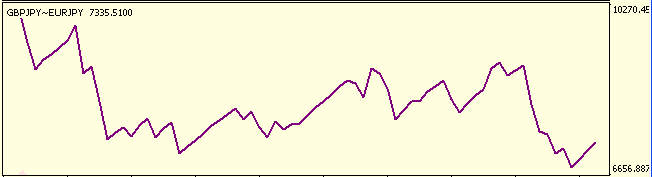Oops! That's ugly. But I think yours will be better.

### Conclusion

Attached files |
performance.mq4 (5.71 KB)
symbol-D1.mq4 (8.23 KB)
VirtualHedge.mq4 (24.81 KB)
Last comments | Go to discussion (7)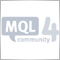| 2 Dec 2009 at 09:47

Now i am working on using a seperate platform to code this strat tester, since we are just interested in the end results, its relatively easier to code everything into 1 program as oppose to multiple programs (EAs) .So i was thinking we might not neccesarily need to use mql to code, but using php and web base html environment for testing, since php can handle reading csv file quite well, and html can create a form to input ur options for the tester;like currency,timeframe, lotsize,..etc.

so what i did was to use the html form to input the parameters,and then use php to execute the simulation for the hedge, and the code is less complicated..basically read from 2 csv history files and compare..repeat till condition is met...| 16 Jan 2010 at 06:31
senshine:

Now i am working on using a seperate platform to code this strat tester, since we are just interested in the end results, its relatively easier to code everything into 1 program as oppose to multiple programs (EAs) .So i was thinking we might not neccesarily need to use mql to code, but using php and web base html environment for testing, since php can handle reading csv file quite well, and html can create a form to input ur options for the tester;like currency,timeframe, lotsize,..etc.

so what i did was to use the html form to input the parameters,and then use php to execute the simulation for the hedge, and the code is less complicated..basically read from 2 csv history files and compare..repeat till condition is met...

I really want to see the results of that outstanding thinking.

Miguel| 25 Dec 2010 at 09:00

Would this work for for smaller timeframe like 1 min?| 6 Jul 2012 at 16:50
Many thanks.......| 16 Aug 2012 at 21:30
this code not work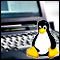Trading Using Linux

The article describes how to use indicators to watch the situation on financial markets online.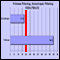Filtering by History

The article describes the usage of virtual trading as an integral part of trade opening filter.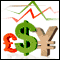Practical Application of Cluster Indicators in FOREX

Cluster indicators are sets of indicators that divide currency pairs into separate currencies. Indicators allow to trace the relative currency fluctuation, determine the potential of forming new currency trends, receive trade signals and follow medium-term and long-term positions.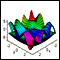Three-Dimensional Graphs - a Professional Tool of Market Analyzing

In this article we will write a simple library for the construction of 3D graphs and their further viewing in Microsoft Excel. We will use standard MQL4 options to prepare and export data into *.csv file.